# Physics Assignment Help With Some Common Forces## Some Common Forces

There are, basically, five forces which we commonly encounter in mechanics problems, namely the weight, the normal forces, the friction force, the tension, the spring force.

### Weight

Weight is the force with which the earth attracts other bodies. It is also called the force of gravity or the gravitational force. It acts upon all the bodies near the earth. If they do not fall to the earth, then their motion is restricted by certain other bodies : a support, string, spring, etc. Bodies that restrict the motion of other bodies are called constraints. These bodies restrict the motion of the given bodies, impeding free motion. For example, the surface of the table is the constraint for all objects lying on it, the floor serves as the constraint for the table, etc.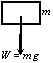A block of mass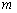experiences the force of gravity called weight.The normal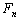and tangential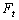components of the contact forces shown on the block and the surface.

### Contact Forces

Whenever two surfaces are in contact they exert forces on each other. Such forces are known as contact forces. It is convenient to resolve these contact forces into components, one parallel to the contact surface, the other perpendicular to that surface.
The normal force is the component of the contact force that is perpendicular to the surface. It is a measure of how strongly the surface in contact is pressed together. Push your hand straight down on the table or desk. The force you feel resisting your push is the normal force of the table pushing up on your hand.

### Frictional Forces

The component of the contact force parallel to the contact surface is called the frictional force. The direction of the frictional force is opposite to the relative motion (or attempted motion) of the two surfaces in contact.

### Tension

The force exerted by the end of a taut string, rope or wire is called the tension. The direction of this force is such that the string pulls the object to which it is attached. It is difficult to push with a string.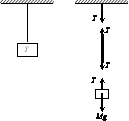### Spring Force

When a spring is deformed (stretched or compressed), it resists the deformation by generating a force called the spring force.
The magnitude of spring force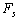is proportional to the deformation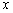as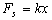where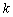is called the stiffness constant or spring constant. In the vector form, it may be written as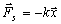The negative sign indicates that the spring force is always opposite to the direction of deformation.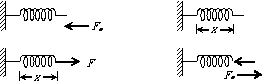### Assignment Help For Some Common Forces

assignmenthelp.net provides best Online Assignment Help service in Physics for all standards. Our Tutor provide their high quality and optimized Tutorial help to fulfill all kind of need of Students.